Test: Area And Perimeter - 1

# Test: Area And Perimeter - 1

Test Description

## 10 Questions MCQ Test Mathematics for Class 4: NCERT | Test: Area And Perimeter - 1

Test: Area And Perimeter - 1 for Class 4 2023 is part of Mathematics for Class 4: NCERT preparation. The Test: Area And Perimeter - 1 questions and answers have been prepared according to the Class 4 exam syllabus.The Test: Area And Perimeter - 1 MCQs are made for Class 4 2023 Exam. Find important definitions, questions, notes, meanings, examples, exercises, MCQs and online tests for Test: Area And Perimeter - 1 below.
Solutions of Test: Area And Perimeter - 1 questions in English are available as part of our Mathematics for Class 4: NCERT for Class 4 & Test: Area And Perimeter - 1 solutions in Hindi for Mathematics for Class 4: NCERT course. Download more important topics, notes, lectures and mock test series for Class 4 Exam by signing up for free. Attempt Test: Area And Perimeter - 1 | 10 questions in 20 minutes | Mock test for Class 4 preparation | Free important questions MCQ to study Mathematics for Class 4: NCERT for Class 4 Exam | Download free PDF with solutions
 1 Crore+ students have signed up on EduRev. Have you?
Test: Area And Perimeter - 1 - Question 1

### Direction: In each of the following questions, 1 cm square grid is given. Based on this information answer the following questions. The area of given shaded shape is ________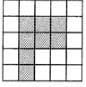Test: Area And Perimeter - 1 - Question 2

### Direction: Timsy is drawing some figures of given area. Find the odd one out in the following questions: Area = 6 square cm.

Detailed Solution for Test: Area And Perimeter - 1 - Question 2

Option d gives a rectangle whose sides are 4 cm and 2 cm, so area of such rectangle will be 4 x2 = 8 cm2

Test: Area And Perimeter - 1 - Question 3

### Direction: In each of the following questions, 1 cm square grid is given. Based on this information answer the following questions. Which among the following square is correctly represent the area of a shape of 24 cm2? (Note that each square is of length 1 cm)

Test: Area And Perimeter - 1 - Question 4

Direction: Find the approximate area of each of the following irregular shapes which are drawn on a graph sheet of 1 cm square grid.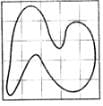Test: Area And Perimeter - 1 - Question 5

Direction: Find the approximate area of each of the following irregular shapes which are drawn on a graph sheet of 1 cm square grid.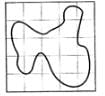Test: Area And Perimeter - 1 - Question 6

Direction: Find the approximate area of each of the following irregular shapes which are drawn on a graph sheet of 1 cm square grid.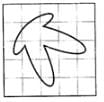Test: Area And Perimeter - 1 - Question 7

Direction: Find the approximate area of each of the following irregular shapes which are drawn on a graph sheet of 1 cm square grid.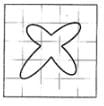Test: Area And Perimeter - 1 - Question 8

Direction: Find the approximate area of each of the following irregular shapes which are drawn on a graph sheet of 1 cm square grid.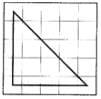Test: Area And Perimeter - 1 - Question 9

Find the area of the triangle whose base is 5 cm and height is 7 cm.

Test: Area And Perimeter - 1 - Question 10

Breadth of a rectangle is 5 cm less than length. If breadth of the rectangle is 13 cm, find the area of the rectangle.

## Mathematics for Class 4: NCERT

24 videos|44 docs|32 tests
 Use Code STAYHOME200 and get INR 200 additional OFF Use Coupon Code
Information about Test: Area And Perimeter - 1 Page
In this test you can find the Exam questions for Test: Area And Perimeter - 1 solved & explained in the simplest way possible. Besides giving Questions and answers for Test: Area And Perimeter - 1, EduRev gives you an ample number of Online tests for practice

## Mathematics for Class 4: NCERT

24 videos|44 docs|32 tests